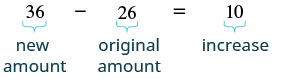## Finding Percent Increase and Percent Decrease

### Learning Outcomes

• Find percent increase given two values
• Find percent decrease given two values

People in the media often talk about how much an amount has increased or decreased over a certain period of time. They usually express this increase or decrease as a percent.

To find the percent increase, first we find the amount of increase, which is the difference between the new amount and the original amount. Then we find what percent the amount of increase is of the original amount.

### Find Percent Increase

Step 1. Find the amount of increase.

• $\text{increase}=\text{new amount}-\text{original amount}$

Step 2. Find the percent increase as a percent of the original amount.

### example

In $2011$, the California governor proposed raising community college fees from $\text{\26}$ per unit to $\text{\36}$ per unit. Find the percent increase. (Round to the nearest tenth of a percent.)

Solution

 What are you asked to find? the percent increase Choose a variable to represent it. Let $p=$ percent. Find the amount of increase.Find the percent increase. The increase is what percent of the original amount? Translate to an equation.Divide both sides by $26$. ${\Large\frac{10}{26}}={\Large\frac{26p}{26}}$ Round to the nearest thousandth. $0.385=p$ Convert to percent form. $\text{38.5%}=p$ Write a complete sentence. The new fees represent a $38.5\text{%}$ increase over the old fees.

### try it

Finding the percent decrease is very similar to finding the percent increase, but now the amount of decrease is the difference between the original amount and the final amount. Then we find what percent the amount of decrease is of the original amount.

### Find percent decrease

1. Find the amount of decrease.
• $\text{decrease}=\text{original amount}-\text{new amount}$
2. Find the percent decrease as a percent of the original amount.

### example

The average price of a gallon of gas in one city in June $2014$ was $\text{\3.71}$. The average price in that city in July was $\text{\3.64}$. Find the percent decrease.

### try it

In the following video we show more examples of how to find percent increase and decrease.